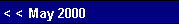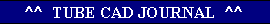First we find Rk:     Rk = Vb/2Iq - rp                   mu + 1,substituting variables     Rk = 200/(2x0.007) - 3300                            33 +1     Rk = 323.Next we find R2,     R1 = Vb / 4Iq - RL - rp                       mu +1,     R1 = 200 / (4x0.007) - 300 - 3300                                    33 + 1     R1 = 104thus,     R2 =  Rk - R1     R2 =  323 - 104     R2 =  219Next we find the peak current Ipk,     Ipk = Vb / 2(rp + (mu +1 )Rl + RL)     Ipk = 200 / 2(3300 + (33 + 1)104 + 300)     Ipk = 0.014 A,and as      2Iq = 0.014 A,we are still on the mark.    The math gets quite thick here, so let's stop at an approximation; the effective Gm of the top triode is given roughly by:

SRPP Series Voltage Regulator
An alternate use of the SRPP topology can retain many of the advantages of the "over-designed" version of the circuit. Remember, when we made the value of resistor Rak very large, we seemingly achieved high gain and low Zo, but at closer examination, we saw that we had reduced the amount of symmetrical current swing into the load impedance. But what if we do not need symmetrical current to swing into the load?
The unspoken assumption was that music was the intended signal, which can be approximated by a sine wave. But what if the signal was not symmetrical? What if we only needed to amplify the top half of the sine wave? In this case the large voltage drop across Rak could be used to drive the top triode into greater conduction without risking turning off the top triode during negative current swings, as there would not be any.
A series voltage regulator is much like one half of a Class AB amplifier. In both circuits there is an asymmetry in current conduction: the devices are driven further in the on direction than the off direction. An output device in a Class AB amplifier sees a small idle current and an input signal that alternately drives the device into heavy conduction and into cutoff. The series regulator's pass device sees a correction signal that drives the device into heavy conduction and then back to idle level and occasionally into cutoff.  And it is this asymmetry in current conduction that we can exploit with an over-designed SRPP, as usually a series voltage regulator is required to push the output voltage up more than it is required to pull it down. (If a reactive load presents a positive pulse to the output of the series regulator, it can only respond by ceasing conduction through its pass device. In this case, a shunt regulator is better suited because it is like a Class A SE amplifier: by running at a much higher idle current, it can push up its output voltage by conducting less and pull it down by conducting more.)

mu

Gm´@(mu + 1)R1+rp+RL

33

Gm´@(33 + 1)104 + 3300 + 300

Gm´= 4.62 mA/v
and
216 = 1/0.00462
which is fairly close to the 219 value of resistor R2. In the design example, we have come close to meeting both goals: a peak positive current swing of 2Iq (14 mA) and the top tube's effective Gm equal to the inverse of resistor R2. (Had we used a higher ohmage load, the Rak would not have as closely matched 1/Gm.)pg. 14
 www.tubecad.com   Copyright © 2000 GlassWare. All Rights Reserved